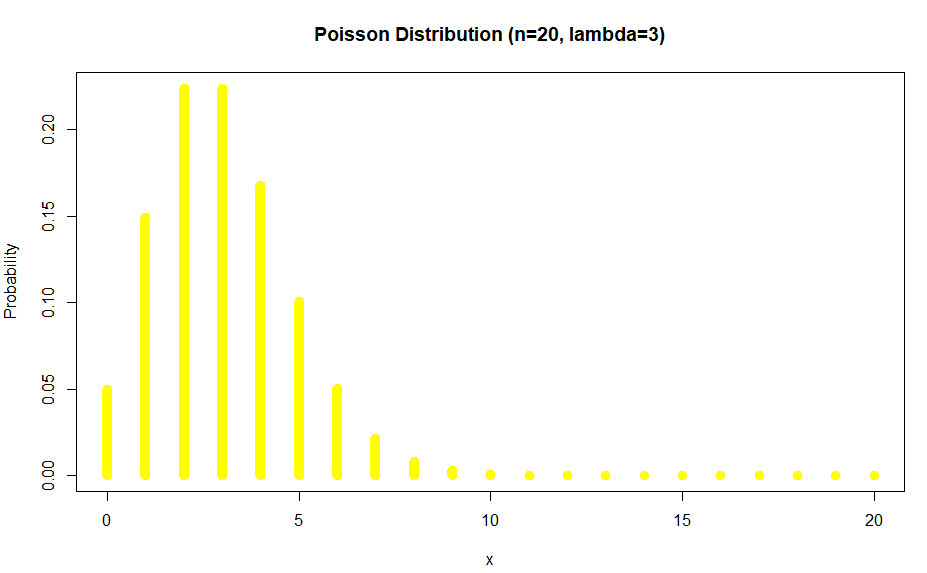# Poisson distribution in R | Example

Poisson distribution is discrete distribution that describes the number of events occurring in a fixed time interval or region of opportunity in general case.

Requires only one parameter $\lambda$ also know as the expected number of events.

This $\lambda$ is the number of events that will occur in this fixed time interval or région d’opportunités.

Support of this function is 0 and $\infty$, or it is bounded on the interval $[0,\infty)$.

As a difference to the binomial distribution, the Poisson distribution goes to the $\infty$.

## Pre requisites

• Constant number of events in constant time interval
• Events occur in sequence (timeline)
• The occurrence of one event doesn’t affect the subsequent event (independence)

## PMF

The probability mass function $f$:

$$f(x; \lambda) = \mathbb{P}(X=x)=\frac{\mathbf{e}^{-\lambda} \lambda^{x}}{x !}$$

where $x \in N_0= {0,1,2, …, \infty}$ is the support.

## CDF

$\mathbb{P}(\mathbf{X} \leq \mathbf{x})=\Large \frac{\Gamma(\lfloor x+1\rfloor, \lambda)}{\lfloor x !\rfloor}$

## Moments

$\mathbb{E}(X)=\lambda$

$\operatorname{var}(X)=\lambda$

## R code to use Poisson distribution

We can use the following R functions for Poisson distribution calculus:

• dpois(x, lambda, log = FALSE)
• ppois(q, lambda, lower.tail = TRUE, log.p = FALSE)
• qpois(p, lambda, lower.tail = TRUE, log.p = FALSE)
• rpois(n, lambda)

These are density, distribution function, quantile function and random generation for the Poisson distribution with parameter $\lambda$.

Example: For $\lambda=3$ plot the Poisson distribution for x={0, 1,…, 20} interval.

x <- 0:20
plot(success,dpois(x,lambda=3),
type='h',
main='Poisson Distribution (n=20, lambda=0.3)',
ylab='Probability',
xlab ='# Successes',
col='yellow',
lwd=10)To get the probability at x=0 we would ask: ppois(0,lambda=3):

Out:

0.04978707


To get the probability at $x=1$ we may ask ppois(1,lambda=3)-ppois(0,lambda=3):

Out:

0.1493612


Of course dpois(x,lambda=3) would aslo give us: Out:

4.978707e-02
1.493612e-01
2.240418e-01
...


Which is exactly what we showed.

Example: Different values of lambdatags: poisson & category: r